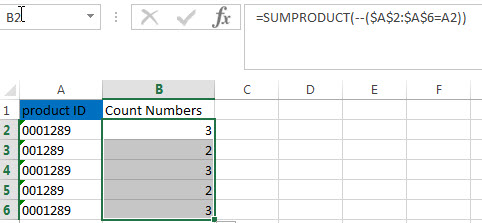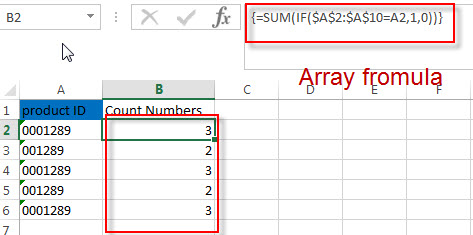# How to Count Numbers with Leading Zeros

This post explains that how to count numbers with leading zeros as Text format in excel. How to count numeric format strings with a different number of leading zeros using excel formula.

## Count Strings with Leading Zeros

Suppost you have a list of product ID in your worksheet, and the product ID contains the different number of leading zeros in the front of string. And if you use the COUNTIF function to count numbers with leading zeros, but it will remove those leading zeros, it means that 1289 and 001289 are the same strings.

So how to preserve the leading zeros while counting numbers. And you can use another Excel function named as SUMPRODUCT.

For example, you want to count strings with leading zeros in the range A2:A6, you can write down the following formula based on the SUMPRODUCT function.

`=SUMPRODUCT(--(\$A\$2:\$A\$6=A2))`

You can enter this formula into Cell B2, then press Enter key. Then you can drag AutoFill Handle down to other cells to apply this formula.You can also use another excel array formula to count strings with leading zeros as follows:

`=SUM(IF(\$A\$2:\$A\$10=A2,1,0))`Note: when you enter this formula into Cell B2, you still need to press Ctrl+Shift+Enter keys to convert this formula as Array formula.

### Related Functions

• Excel SUMPRODUCT function
The Excel SUMPRODUCT function multiplies corresponding components in the given one or more arrays or ranges, and returns the sum of those products.The syntax of the SUMPRODUCT function is as below:= SUMPRODUCT (array1,[array2],…)…
• Excel COUNTIF function
The Excel COUNTIF function will count the number of cells in a range that meet a given criteria. This function can be used to count the different kinds of cells with number, date, text values, blank, non-blanks, or containing specific characters.etc.= COUNTIF (range, criteria)…
• Excel IF function
The Excel IF function perform a logical test to return one value if the condition is TRUE and return another value if the condition is FALSE. The IF function is a build-in function in Microsoft Excel and it is categorized as a Logical Function.The syntax of the IF function is as below:= IF (condition, [true_value], [false_value])….
• Excel SUM function
The Excel SUM function will adds all numbers in a range of cells and returns the sum of these values. You can add individual values, cell references or ranges in excel.The syntax of the SUM function is as below:= SUM(number1,[number2],…)…

### Related Posts

• How to insert leading zeros to number or text
Assuming that you want to insert leading zeros  to number in Cell A2, then you can try to use the TEXT function to create an excel formula. You can also use the CONCATENATE function to add the specific digit of leading zeros into each number…
• How to keep or remove leading zeros
If you want to keep the leading zeros as you type in a cell, you need to change the cell format to Text before you type the zip code number.You can remove or delete leading zeros in front of the cells with excel VBA macro…
• Count the number of words in a cell
If you want to count the number of words in a single cell, you can create an excel formula based on the IF function, the LEN function, the TRIM function and the SUBSTITUTE function. ..

Related Posts

Calculate Win Loss Tie

Suppose you got a task to calculate the win, loss, and tie totals; what would you do? If you are new to Ms Excel and don't have enough experience with it, then you might do this task manually but let ...

Count Cells that are Case Sensitive

If you are a frequent user of Microsoft Excel, you may have come across situations where you needed to add or count the cells that were case-sensitive. If you have done this task manually, I would like to point out ...

Check Dates in chronological order

Assume you have a date list that has different date formats, as seen in the accompanying picture. In this instance, Excel's Sort function will fail to sort them appropriately. However, you may convert all various date formats to a particular ...

Case Sensitive Lookup with SUMPRODUCT and EXACT

Today, we will show you how to use SUMPRODUCT and EXACT to perform a case sensitive exact match. In this article, we provide a simple example to calculate bonus for employees whose names are case-sensitive. If you meet similar scenarios ...

Average per Week by Formula in Excel

We usually apply AVERAGE function or relevant functions to return average directly in Excel worksheet. But in some situations, only applying average relevant functions cannot figure out our problem. Sometimes we can create a formula with functions and mathematical operation ...

How to Sum if Equal to Many Items or A Range in Excel

If we want to sum numbers from a range with criteria, we often select SUMIF of SUMIFS functions as the first choice to create a formula. The criteria can be a number or an array or a collection of some ...

How to Sum if Equal to X or Y in Excel

In daily work, if we want to sum numbers from a range, and only sum the numbers which being equal to X or Y in the range, we can create a formula with Excel build-in functions to get the result. ...

How To Sum the Largest N Values in Excel

Sometimes we may want to sum the largest N numbers or top N numbers in a range. In this article, we will show you the method of “SUM the Largest N Numbers” by a simple formula which consist of SUMPRODUCT ...

How to Sum for Cell Contains Formula Only in Excel

Sometimes values are created by formulas in cells. If we want to sum values which are created by formulas from a range, but some values which are hardcoded also list in the same range, how can we filter out matched ...

How to Sum the Smallest N Values in Excel

Sometimes we may want to sum the first smallest N numbers in a range in Excel. In this article, we will show you the method of “SUM the Smallest N Numbers” by a simple formula which consist of SUMPRODUCT and ...

Sidebar